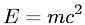Equations > Physics > Modern Physics > Einstein's relativistic mass-energy relation

### Einstein's relativistic mass-energy relationLatex Code:

MathML Code:

 $E={\mathrm{mc}}^{2}$

MathType 5.0: